# SI Controller

Spark-ignition engine controller that uses the driver torque request

•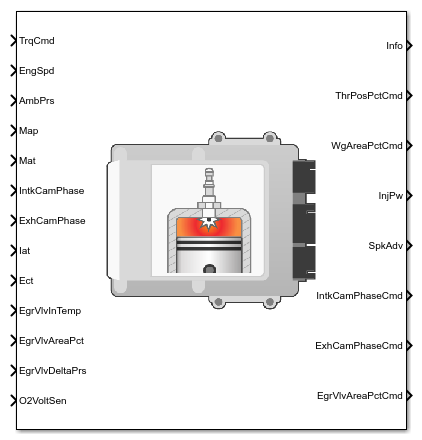Libraries:
Powertrain Blockset / Propulsion / Combustion Engine Controllers

## Description

The SI Controller block implements a spark-ignition (SI) controller that uses the driver torque request to calculate the open-loop air, fuel, and spark actuator commands that are required to meet the driver demand.

You can use the SI Controller block in engine control design or performance, fuel economy, and emission tradeoff studies. The core engine, throttle, and turbocharger wastegate subsystems require the commands that are output from the SI Controller block.

The block uses the commanded torque and engine speed to determine these open-loop actuator commands:

• Throttle position percent

• Wastegate area percent

• Injector pulse-width

• Intake cam phaser angle

• Exhaust cam phaser angle

• Exhaust gas recirculation (EGR) valve area percent

The SI Controller block has two subsystems:

• The `Controller` subsystem — Determines the commands based on the commanded torque, measured engine speed, and estimated cylinder air mass.

• The `Estimator` subsystem — Determines the estimated air mass flow, torque, and exhaust gas temperature from intake manifold gas pressure, intake manifold gas temperature, engine speed, and cam phaser positions.

The figure illustrates the signal flow.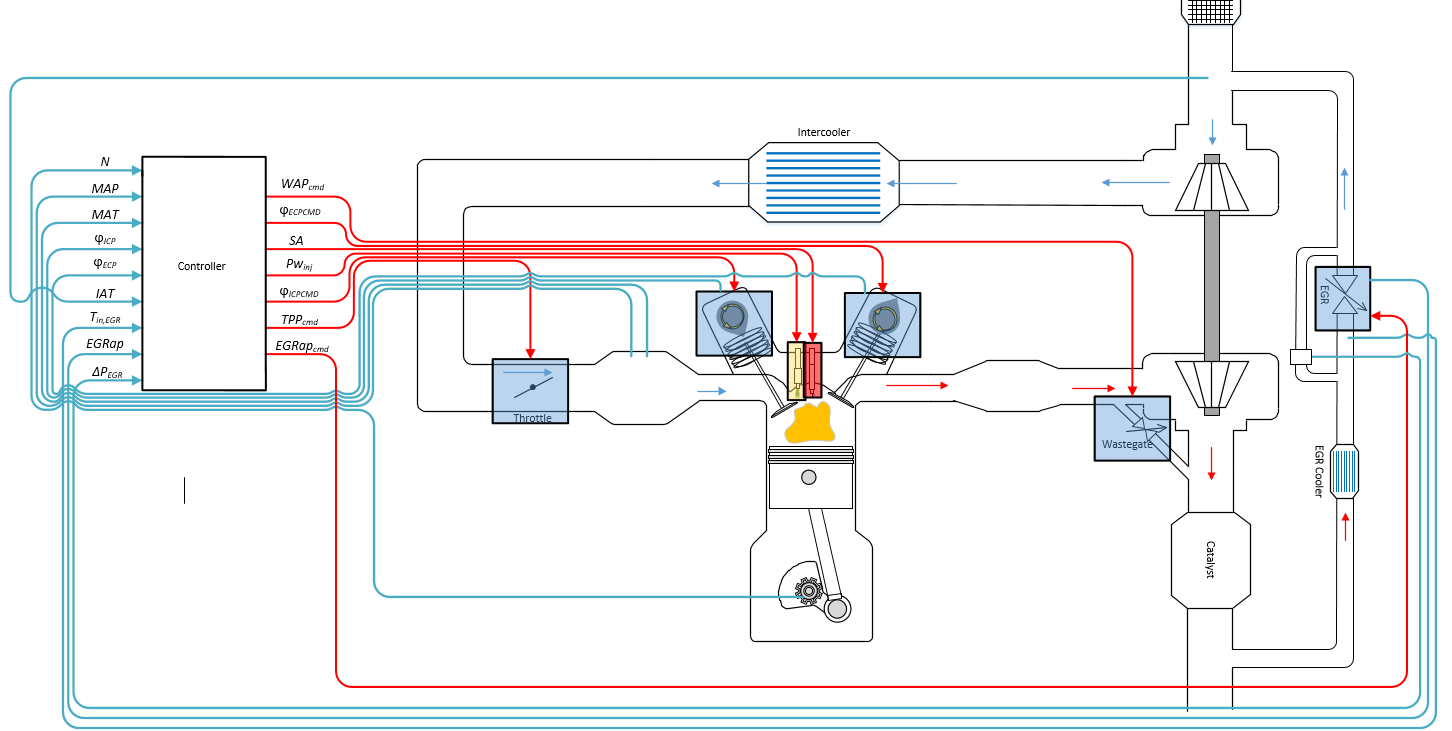The figure uses these variables.

 N Engine speed MAP Cycle average intake manifold pressure IAT Intake air temperature Tin,EGR Temperature at EGR valve inlet MAT Cycle average intake manifold gas absolute temperature ${\phi }_{ICP}$, ${\phi }_{ICPCMD}$ Intake cam phaser angle and intake cam phaser angle command, respectively ${\phi }_{ECP}$, ${\phi }_{ECPCMD}$ Exhaust cam phaser angle and exhaust cam phaser angle command, respectively EGRap, EGRapcmd EGR valve area percent and EGR valve area percent command, respectively ΔPEGR Pressure difference at EGR valve inlet and outlet WAPcmd Turbocharger wastegate area percent command SA Spark advance $P{w}_{inj}$ Fuel injector pulse-width TPPcmd Throttle position percent command

The Model-Based Calibration Toolbox™ was used to develop the tables that are available with the Powertrain Blockset™.

### Controller

Air

The block determines the commanded engine load (that is, normalized cylinder air mass) from a lookup table that is a function of commanded torque and measured engine speed.

`${L}_{cmd}={f}_{Lcmd}\left({T}_{cmd},N\right)$`

To achieve the commanded load, the controller sets the throttle position percent and turbocharger wastegate area percent using feed forward lookup tables. The lookup tables are functions of the commanded load and measured engine speed.

`$TA{P}_{cmd}={f}_{TAPcmd}\left({L}_{cmd},N\right)$`

`$TP{P}_{cmd}={f}_{TPPcmd}\left(TA{P}_{cmd}\right)$`

`$WA{P}_{cmd}={f}_{WAPcmd}\left({L}_{cmd},N\right)$`

To determine the cam phaser angle commands, the block uses lookup tables that are functions of estimated engine load and measured engine speed.

`${\phi }_{ICPCMD}={f}_{ICPCMD}\left({L}_{est},N\right)$`

`${\phi }_{ECPCMD}={f}_{ECPCMD}\left({L}_{est},N\right)$`

The block calculates the desired engine load using this equation.

`${L}_{est}=\frac{Cps{R}_{air}{T}_{std}{\stackrel{˙}{m}}_{air,est}}{{P}_{std}{V}_{d}N}$`

The equations use these variables.

 Lest Estimated engine load Lcmd Commanded engine load N Engine speed Tcmd Commanded engine torque TAPcmd Throttle area percent command TPPcmd Throttle position percent command WAPcmd Turbocharger wastegate area percent command $Cps$ Crankshaft revolutions per power stroke ${P}_{std}$ Standard pressure ${T}_{std}$ Standard temperature ${R}_{air}$ Ideal gas constant for air and burned gas mixture ${V}_{d}$ Displaced volume ${\stackrel{˙}{m}}_{air,est}$ Estimated engine air mass flow

The controller subsystem uses these lookup tables for the air calculations.

• The throttle area percent command lookup table, ${f}_{TAPcmd}$, is a function of commanded load and engine speed

`$TA{P}_{cmd}={f}_{TAPcmd}\left({L}_{cmd},N\right)$`

where:

• TAPcmd is throttle area percentage command, in percent.

• Lcmd=L is commanded engine load, dimensionless.

• N is engine speed, in rpm.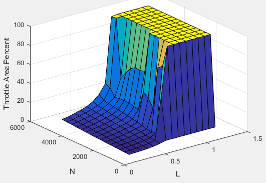• To account for the non-linearity of the throttle position to throttle area, the throttle position percent lookup table linearizes the open-loop air mass flow control.

The throttle position percent command lookup table, ${f}_{TPPcmd}$, is a function of the throttle area percentage command

`$TP{P}_{cmd}={f}_{TPPcmd}\left(TA{P}_{cmd}\right)$`

where:

• TPPcmd is throttle position percentage command, in percent.

• TAPcmd is throttle area percentage command, in percent.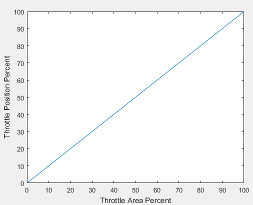• The wastegate area percent command lookup table, ${f}_{WAPcmd}$, is a function of the commanded engine load and engine speed

`$WA{P}_{cmd}={f}_{WAPcmd}\left({L}_{cmd},N\right)$`

where:

• WAPcmd is wastegate area percentage command, in percent.

• Lcmd=L is commanded engine load, dimensionless.

• N is engine speed, in rpm.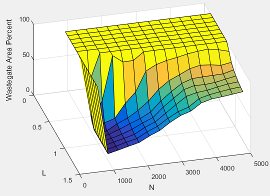• The commanded engine load lookup table, ${f}_{Lcmd}$, is a function of the commanded torque and engine speed

`${L}_{cmd}={f}_{Lcmd}\left({T}_{cmd},N\right)$`

where:

• Lcmd=L is commanded engine load, dimensionless.

• Tcmd is commanded torque, in N·m.

• N is engine speed, in rpm.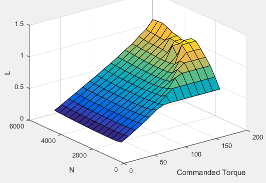• The intake cam phaser angle command lookup table, ${f}_{ICPCMD}$, is a function of the engine load and engine speed

`${\phi }_{ICPCMD}={f}_{ICPCMD}\left({L}_{est},N\right)$`

where:

• ${\phi }_{ICPCMD}$ is commanded intake cam phaser angle, in degrees crank advance.

• Lest=L is estimated engine load, dimensionless.

• N is engine speed, in rpm.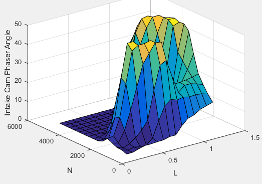• The exhaust cam phaser angle command lookup table, ${f}_{ECPCMD}$, is a function of the engine load and engine speed

`${\phi }_{ECPCMD}={f}_{ECPCMD}\left({L}_{est},N\right)$`

where:

• ${\phi }_{ECPCMD}$ is commanded exhaust cam phaser angle, in degrees crank retard.

• Lest=L is estimated engine load, dimensionless.

• N is engine speed, in rpm.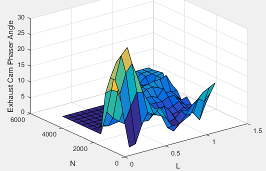EGR

EGR is typically expressed as a percent of total intake port flow.

`$EG{R}_{pct}=100\frac{{\stackrel{˙}{m}}_{EGR}}{{\stackrel{˙}{m}}_{EGR}+{\stackrel{˙}{m}}_{air}}$`

To calculate the EGR area percent command, the block uses equations and a lookup table.

 Equations `$\begin{array}{l}{\stackrel{˙}{m}}_{EGRstd,cmd}={\stackrel{˙}{m}}_{EGR,cmd}\frac{{P}_{std}}{{P}_{in,EGR}}\sqrt{\frac{{T}_{in,EGR}}{{T}_{std}}}\\ \\ {\stackrel{˙}{m}}_{EGRstd,max}={f}_{EGRstd,max}\left(\frac{{P}_{out,EGR}}{{P}_{in,EGR}}\right)\\ \\ {\stackrel{˙}{m}}_{EGR,cmd}=EG{R}_{pct,cmd}{\stackrel{˙}{m}}_{intk,est}\end{array}$` Lookup table The EGR area percent command, EGRapcmd, lookup table is a function of the normalized mass flow and pressure ratio `$EGRa{p}_{cmd}={f}_{EGRap,cmd}\left(\frac{{\stackrel{˙}{m}}_{EGRstd,cmd}}{{\stackrel{˙}{m}}_{EGRstd,max}},\frac{{P}_{out,EGR}}{{P}_{in,EGR}}\right)$` where: EGRapcmd is commanded EGR area percent, dimensionless.$\frac{{\stackrel{˙}{m}}_{EGRstd,cmd}}{{\stackrel{˙}{m}}_{EGRstd,max}}$ is the normalized mass flow, dimensionless. $\frac{{P}_{out,EGR}}{{P}_{in,EGR}}$ is the pressure ratio, dimensionless.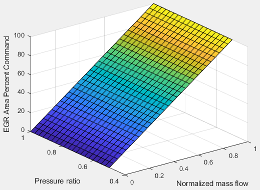The equations and table use these variables.

 EGRap, EGRapcmd EGR valve area percent and EGR valve area percent command, respectively EGRpct,cmd EGR percent command ${\stackrel{˙}{m}}_{EGRstd,cmd}$ Commanded standard mass flow ${\stackrel{˙}{m}}_{EGRstd,max}$ Maximum standard mass flow ${\stackrel{˙}{m}}_{EGR,cmd}$ Commanded mass flow ${\stackrel{˙}{m}}_{intk,est}$ Estimated intake port mass flow Tstd, Pstd Standard temperature and pressure Tin,EGR Temperature at EGR valve inlet Pout,EGR, Pin,EGR Pressure at EGR valve inlet and outlet, respectively

Fuel

The air-fuel ratio (AFR) impacts three-way-catalyst (TWC) conversion efficiency, torque production, and combustion temperature. The engine controller manages AFR by commanding injector pulse-width from a desired relative AFR. The relative AFR, ${\lambda }_{cmd}$, is the ratio between the commanded AFR and the stoichiometric AFR of the fuel.

`${\lambda }_{cmd}=\frac{AF{R}_{cmd}}{AF{R}_{stoich}}$`

`$AF{R}_{cmd}=\frac{{\stackrel{˙}{m}}_{air,est}}{{\stackrel{˙}{m}}_{fuel,cmd}}$`

The SI Controller block accounts for the extra fuel delivered to the SI engine during startup. If the engine speed is greater than the startup engine cranking speed, the SI Controller block enriches the optimal AFR, lambda, with an exponentially decaying delta lambda. To initialize the delta lambda, the block uses the engine coolant temperature at startup. The delta lambda exponentially decays to zero based on a time constant that is a function of the engine coolant temperature.

You can configure the block for open-loop and closed-loop AFR control.

To

Use

Controls > Fuel > Closed-loop feedback Parameter Setting

• Assess the dynamic and steady-state accuracy of the controller airflow estimation and fuel delivery.

(default) Open-loop control

`off`

• Hold the average AFR close to stoichiometric AFR to maintain a high TWC conversion efficiency.

Closed-loop control

`on`

Open-Loop Control

To create an input port for the commanded AFR (lambda), on the Controls > Fuel > Open-loop fuel pane, select Input lambda.

You can manually tune the catalyst for maximum efficiency during open-loop AFR control with or without dither. If you want to implement dither during open-loop control, on the Fuel tab, on the Closed-loop fuel pane, select .

By default, the block is configured to use a lookup table for the commanded AFR.

The commanded lambda, ${\lambda }_{cmd}$, lookup table is a function of estimated engine load and measured engine speed

`${\lambda }_{cmd}={f}_{\lambda cmd}\left({L}_{est},N\right)$`

where:

• ${\lambda }_{cmd}$ is commanded relative AFR, dimensionless.

• Lest=L is estimated engine load, dimensionless.

• N is engine speed, in rpm.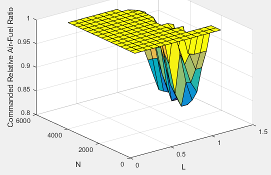The block calculates the estimated fuel mass flow rate using the commanded lambda, ${\lambda }_{cmd}$, stoichiometric AFR, and estimated air mass flow rate.

`${\stackrel{˙}{m}}_{fuel,cmd}=\frac{{\stackrel{˙}{m}}_{air,est}}{AF{R}_{cmd}}=\frac{{\stackrel{˙}{m}}_{air,est}}{{\lambda }_{cmd}AF{R}_{stoich}}$`

The block assumes that the battery voltage and fuel pressure are at nominal settings where pulse-width correction is not necessary. The commanded fuel injector pulse-width is proportional to the fuel mass per injection. The fuel mass per injection is calculated from the commanded fuel mass flow rate, engine speed, and the number of cylinders.

Closed-Loop Control

TWC converters are most efficient when the exhaust AFR is near the stoichiometric AFR, where the air and fuel burn most completely. Around this ideal point, the AFR is within the catalyst window in which the catalyst is most efficient at converting carbon monoxide, hydrocarbons, and nitrogen oxides to non-harmful exhaust products. Empirical studies show that oscillating the AFR around stoichiometry at an optimized AFR frequency, amplitude, and bias widens the TWC window, increasing catalyst conversion efficiency in the presence of unavoidable disturbances.

To keep production hardware costs down, AFR control systems include inexpensive switching oxygen sensors positioned in the engine exhaust stream upstream and downstream of the catalyst. The oxygen sensors have a narrow range. Essentially, they switch between too lean (i.e., more air is available than is required to burn the available fuel) and too rich (i.e., more air is available than is required to burn the available fuel).

The block implements a period-based method to control the average AFR at a value within the catalyst window for maximum conversion efficiency. Period-based AFR control is independent of the transport delay across the engine from the fuel injection point to the sensor measurement point. For more information about the method, see Developing a Period-Based Air-Fuel Ratio Controller Using a Low-Cost Switching Sensor.

Spark

Spark advance is the crank angle before top dead center (BTDC) of the power stroke when the spark is delivered. The spark advance has an impact on engine efficiency, torque, exhaust temperature, knock, and emissions.

The spark advance lookup table is a function of estimated load and engine speed.

`$SA={f}_{SA}\left({L}_{est},N\right)$`

where:

• Lest=L is estimated engine load, dimensionless.

• N is engine speed, in rpm.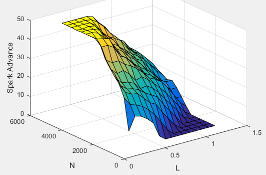The equations use these variables.

 Lest Estimated engine load, based on normalized cylinder air mass N Engine speed ${f}_{SA}$ Lookup table for spark advance N Spark advance

Idle Speed

When the commanded torque is below a threshold value, the idle speed controller regulates the engine speed.

IfIdle Speed Controller
Trqcmd,input < Trqidlecmd,enableEnabled
Trqidlecmd,enableTrqcmd,inputNot enabled

The idle speed controller uses a discrete PI controller to regulate the target idle speed by commanding a torque.

The PI controller uses this transfer function:

`${C}_{idle}\left(z\right)={K}_{p,idle}+{K}_{i,idle}\frac{{t}_{s}}{z-1}$`

The idle speed commanded torque must be less than the maximum commanded torque:

0 ≤ TrqidlecomdTrqidlecmd,max

Idle speed control is active under these conditions. If the commanded input torque drops below the threshold for enabling the idle speed controller (Trqcmd,input < Trqidlecmd,enable), the commanded engine torque is given by:

Trqcmd = max(Trqcmd,input,Trqidlecmd).

The equations use these variables.

 Trqcmd Commanded engine torque Trqcmd,input Input commanded engine torque Trqidlecmd,enable Threshold for enabling idle speed controller Trqidlecmd Idle speed controller commanded torque Trqidlecmd,max Maximum commanded torque Nidle Base idle speed Kp,idle Idle speed controller proportional gain Ki,idle Idle speed controller integral gain

Speed Limiter

To prevent over revving the engine, the block implements an engine speed limit controller that limits the engine speed to the value specified by the Rev-limiter speed threshold parameter on the Controls > Idle Speed tab.

If the engine speed, N, exceeds the engine speed limit, Nlim, the block sets the commanded engine torque to 0.

To smoothly transition the torque command to 0 as the engine speed approaches the speed limit, the block implements a lookup table multiplier. The lookup table multiplies the torque command by a value that ranges from 0 (engine speed exceeds limit) to 1 (engine speed does not exceed the limit).

### Estimator

The estimator subsystem determines the estimated air mass flow, torque, EGR mass flow, and exhaust temperature based on sensor feedback and calibration parameters.

 ${\stackrel{˙}{m}}_{air,est}$ Estimated engine air mass flow Trqest Estimated engine torque Texh,est Estimated engine exhaust temperature ${\stackrel{˙}{m}}_{EGR,est}$ Estimated low-pressure EGR mass flow

Air Mass Flow

To calculate engine air mass flow, configure the SI engine to use either of these air mass flow models.

Air Mass Flow ModelDescription
SI Engine Speed-Density Air Mass Flow Model

Uses the speed-density equation to calculate the engine air mass flow, relating the engine air mass flow to the intake manifold pressure and engine speed. Consider using this air mass flow model in engines with fixed valvetrain designs.

SI Engine Dual-Independent Cam Phaser Air Mass Flow Model

To calculate the engine air mass flow, the dual-independent cam phaser model uses:

• Empirical calibration parameters developed from engine mapping measurements

• Desktop calibration parameters derived from engine computer-aided design (CAD) data

In contrast to typical embedded air mass flow calculations based on direct air mass flow measurement with an air mass flow (MAF) sensor, this air mass flow model offers:

• Elimination of MAF sensors in dual cam-phased valvetrain applications

• Reasonable accuracy with changes in altitude

• Semiphysical modeling approach

• Bounded behavior

• Suitable execution time for electronic control unit (ECU) implementation

• Systematic development of a relatively small number of calibration parameters

To determine the estimated air mass flow, the block uses the intake air mass fraction. The EGR mass fraction at the intake port lags the mass fraction near the EGR valve outlet. To model the lag, the block uses a first order system with a time constant.

`${y}_{intk,EGR,est}=\frac{{\stackrel{˙}{m}}_{EGR,est}}{{\stackrel{˙}{m}}_{intk,est}}\frac{{t}_{s}z}{{\tau }_{EGR}z+{t}_{s}-{\tau }_{EGR}}$`

The remainder of the gas is air.

`${y}_{intk,air,est}=1-{y}_{intk,EGR,est}$`

The equations use these variables.

 yintk,EGR,est Estimated intake manifold EGR mass fraction yintk,air,est Estimated intake manifold air mass fraction ${\stackrel{˙}{m}}_{EGR,est}$ Estimated low-pressure EGR mass flow ${\stackrel{˙}{m}}_{intk,est}$ Estimated intake port mass flow τEGR EGR time constant

Torque

To calculate the brake torque, configure the SI engine to use either of these torque models.

Brake Torque ModelDescription
SI Engine Torque Structure Model

For the structured brake torque calculation, the SI engine uses tables for the inner torque, friction torque, optimal spark, spark efficiency, and lambda efficiency.

If you select Crank angle pressure and torque on the block Torque tab, you can:

• Simulate advanced closed-loop engine controls in desktop simulations and on HIL bench, based on cylinder pressure recorded from a model or laboratory test as a function of crank angle.

• Simulate driveline vibrations downstream of the engine due to high-frequency crankshaft torsionals.

• Simulate engine misfires due to lean operation or spark plug fouling by using the injector pulse width input.

• Simulate cylinder deactivation effect (closed intake and exhaust valves, no injected fuel) on individual cylinder pressures, mean-value airflow, mean-value torque, and crank-angle-based torque.

• Simulate the fuel-cut effect on individual cylinder pressure, mean-value torque, and crank-angle-based torque.

SI Engine Simple Torque Model

For the simple brake torque calculation, the SI engine block uses a torque lookup table map that is a function of engine speed and load.

EGR

The controller estimates low-pressure mass flow, EGR valve inlet pressure, and EGR valve outlet pressure using an algorithm developed by F. Liu and J. Pfeiffer. The estimator requires measured EGR valve differential pressure, EGR valve area percent, intake air temperature, and EGR valve inlet temperature.

To estimate the EGR valve commands, the block uses:

• Equations

`$\begin{array}{l}{\stackrel{˙}{m}}_{air,std}={\stackrel{˙}{m}}_{air,est}\frac{{P}_{std}}{{P}_{amb}}\sqrt{\frac{IAT}{{T}_{std}}}\\ \\ {P}_{in,EGR}={P}_{out,EGR}+\Delta {P}_{EGR}\\ \\ {\stackrel{˙}{m}}_{EGR,est}={\stackrel{˙}{m}}_{EGR,std}\frac{{P}_{in,EGR}}{{P}_{std}}\sqrt{\frac{{T}_{std}}{{T}_{in,EGR}}}\end{array}$`

• Tables

• The EGR valve standard mass flow lookup table is a function of EGR valve area percent and the pressure ratio

`${\stackrel{˙}{m}}_{EGR,std}={f}_{EGR,std}\left(EGRap,\frac{{P}_{out,EGR}}{{P}_{in,EGR}}\right)$`

where:

• ${\stackrel{˙}{m}}_{EGR,std}$ is EGR valve standard mass flow, dimensionless.

• EGRap is EGR valve flow area percent, in percent.

• $\frac{{P}_{out,EGR}}{{P}_{in,EGR}}$ is the pressure ratio, dimensionless.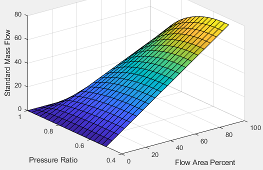• The pressure ratio is a function of the standard mass flow

`$\frac{{P}_{out,EGR}}{{P}_{amb}}={f}_{intksys,pr}\left({\stackrel{˙}{m}}_{air,std}\right)$`

where:

• ${\stackrel{˙}{m}}_{air,std}$ is standard mass flow, in g/s.

• $\frac{{P}_{out,EGR}}{{P}_{amb}}$ is pressure ratio, dimensionless.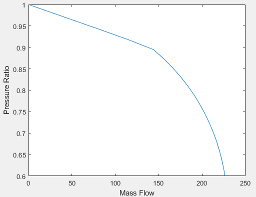The equations use these variables.

 EGRap EGR valve area percent command IAT Intake air temperature ${\stackrel{˙}{m}}_{air,std}$, ${\stackrel{˙}{m}}_{EGR,std}$ Standard air and EGR valve mass flow, respectively ${\stackrel{˙}{m}}_{air,est}$,${\stackrel{˙}{m}}_{EGR,est}$ Estimated air and EGR valve mass flow, respectively Tstd, Pstd Standard temperature and pressure Tamb, Pamb Ambient temperature and pressure ΔPEGR Pressure difference at EGR valve inlet and outlet Tin,EGR, Tout,EGR Temperature at EGR valve inlet and outlet, respectively Pin,EGR, Pout,EGR Pressure at EGR valve inlet and outlet, respectively

Exhaust Temperature

The exhaust temperature lookup table, ${f}_{Texh}$, is a function of engine load and engine speed

`${T}_{exh}={f}_{Texh}\left(L,N\right)$`

where:

• Texh is engine exhaust temperature, in K.

• L is normalized cylinder air mass or engine load, dimensionless.

• N is engine speed, in rpm.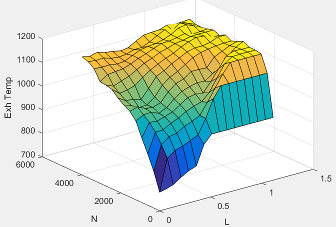## Ports

### Input

expand all

Commanded engine torque, Trqcmd,input, in N·m.

Measured engine speed, N, in rpm.

Measured ambient pressure, ${P}_{Amb}$, in Pa.

Measured intake manifold absolute pressure$MAP$, in Pa.

Measured intake manifold absolute temperature, $MAT$, in K.

Intake cam phaser angle, ${\phi }_{ICP}$, in degCrkAdv, or degrees crank advance.

Exhaust cam phaser angle, ${\phi }_{ECP}$, in degCrkRet, or degrees crank retard.

Intake air temperature, IAT, in K.

Engine cooling temperature, Tcoolant, in K.

EGR valve inlet temperature, Tin,EGR, in K.

EGR valve area percent, EGRap, in %.

EGR valve delta pressure, ΔPEGR, in Pa.

Oxygen sensor voltage for closed-loop air-fuel-ratio (lambda) control, in mV.

To configure the block to use closed-loop air-fuel-ratio control, on the Fuel tab, on the Closed-loop fuel pane, select Closed-loop feedback.

Commanded air-fuel-ratio (lambda), λcmd, dimensionless.

#### Dependencies

To create this port, on the Fuel tab, on the Open-loop fuel pane, select Input lambda.

State of the vehicle ignition switch, dimensionless.

#### Dependencies

To create this port, on the Stop-Start tab, select Enable Engine Stop-Start.

Command to enable or disable the stop-start logic, dimensionless.

#### Dependencies

To create this port, on the Stop-Start tab, select Enable Engine Stop-Start. Select External Enable Port.

### Output

expand all

Bus signal containing these block calculations.

SignalDescriptionVariableUnits

`TrqCmd`

Engine torque

Trqcmd

N·m

`LdCmd`

Lcmd

N/A

`ThrPosCmd`

Throttle area percent command

TAPcmd

%

`WgAreaPctCmd`

Wastegate area percent command

WAPcmd

%

`InjPw`

Fuel injector pulse-width

$P{w}_{inj}$

ms

`SpkAdv`

$SA$

degBTDC

`IntkCamPhaseCmd`

Intake cam phaser angle command

${\phi }_{ICPCMD}$

`ExhCamPhaseCmd`

Exhaust cam phaser angle command

${\phi }_{ECPCMD}$

degCrkRet

`EgrVlvAreaPctCmd`

Exhaust cam phaser angle command

EGRapcmd

%

`FuelMassFlwCmd`

EGR valve area percent command

${\stackrel{˙}{m}}_{fuel,cmd}$

kg/s

`AfrCmd`

Commanded air-fuel ratio

AFRcmdN/A

`EstEngTrq`

Estimated engine torque

Trqest

N·m

`EstNrmlzdAirCharg`

Estimated normalized cylinder air mass

N/A

N/A

`EstIntkPortMassFlw`

Estimated intake port air mass flow rate

${\stackrel{˙}{m}}_{intk,est}$

kg/s

`EstIntkAirMassFlw`

Estimated air mass flow rate

${\stackrel{˙}{m}}_{air,est}$

kg/s

`EstEgrMassFlw`

Estimated low-pressure EGR mass flow rate

${\stackrel{˙}{m}}_{EGR,est}$kg/s

`EstExhManGasTemp`

Estimated exhaust manifold gas temperature

Texh,est

K

`EngRevLimAct`

Flag that indicates if rev-limiter control is active

N/A

N/A

`ClsdLpFuelMult`

Fuel injector pulse-width multiplier for closed-loop AFR control

Pwinj_mult

N/A

Throttle area percent command, TAPcmd.

Wastegate area percent command, WAPcmd.

Fuel injector pulse-width, $P{w}_{inj}$, in ms.

Spark advance, $SA$, in degrees crank angle before top dead center (degBTDC).

Intake cam phaser angle command, ${\phi }_{ICPCMD}$.

Exhaust cam phaser angle command, ${\phi }_{ECPCMD}$.

EGR valve area percent command, EGRapcmd, in %.

## Parameters

expand all

### Configuration

To calculate engine air mass flow, configure the SI engine to use either of these air mass flow models.

Air Mass Flow ModelDescription
SI Engine Speed-Density Air Mass Flow Model

Uses the speed-density equation to calculate the engine air mass flow, relating the engine air mass flow to the intake manifold pressure and engine speed. Consider using this air mass flow model in engines with fixed valvetrain designs.

SI Engine Dual-Independent Cam Phaser Air Mass Flow Model

To calculate the engine air mass flow, the dual-independent cam phaser model uses:

• Empirical calibration parameters developed from engine mapping measurements

• Desktop calibration parameters derived from engine computer-aided design (CAD) data

In contrast to typical embedded air mass flow calculations based on direct air mass flow measurement with an air mass flow (MAF) sensor, this air mass flow model offers:

• Elimination of MAF sensors in dual cam-phased valvetrain applications

• Reasonable accuracy with changes in altitude

• Semiphysical modeling approach

• Bounded behavior

• Suitable execution time for electronic control unit (ECU) implementation

• Systematic development of a relatively small number of calibration parameters

#### Dependencies

The table summarizes the parameter dependencies.

Air Mass Flow Estimation ModelEnables Parameters on Estimation > Air Tab

```Dual Variable Cam Phasing```

Cylinder volume at intake valve close table, f_vivc

Cylinder volume intake cam phase breakpoints, f_vivc_icp_bpt

Cylinder trapped mass correction factor, f_tm_corr

Normalized density breakpoints, f_tm_corr_nd_bpt

Engine speed breakpoints, f_tm_corr_n_bpt

Air mass flow, f_mdot_air

Exhaust cam phase breakpoints, f_mdot_air_ecp_bpt

Trapped mass flow breakpoints, f_mdot_trpd_bpt

Air mass flow correction factor, f_mdot_air_corr

Engine load breakpoints for air mass flow correction, f_mdot_air_corr_ld_bpt

Engine speed breakpoints for air mass flow correction, f_mdot_air_n_bpt

```Simple Speed-Density```

Speed-density volumetric efficiency, f_nv

Speed-density intake manifold pressure breakpoints, f_nv_prs_bpt

Speed-density engine speed breakpoints, f_nv_n_bpt

To calculate the brake torque, configure the SI engine to use either of these torque models.

Brake Torque ModelDescription
SI Engine Torque Structure Model

For the structured brake torque calculation, the SI engine uses tables for the inner torque, friction torque, optimal spark, spark efficiency, and lambda efficiency.

If you select Crank angle pressure and torque on the block Torque tab, you can:

• Simulate advanced closed-loop engine controls in desktop simulations and on HIL bench, based on cylinder pressure recorded from a model or laboratory test as a function of crank angle.

• Simulate driveline vibrations downstream of the engine due to high-frequency crankshaft torsionals.

• Simulate engine misfires due to lean operation or spark plug fouling by using the injector pulse width input.

• Simulate cylinder deactivation effect (closed intake and exhaust valves, no injected fuel) on individual cylinder pressures, mean-value airflow, mean-value torque, and crank-angle-based torque.

• Simulate the fuel-cut effect on individual cylinder pressure, mean-value torque, and crank-angle-based torque.

SI Engine Simple Torque Model

For the simple brake torque calculation, the SI engine block uses a torque lookup table map that is a function of engine speed and load.

#### Dependencies

The table summarizes the parameter dependencies.

Torque Estimation ModelEnables Parameters on Estimation > Torque Tab

```Torque Structure```

Inner torque table, f_tq_inr

Friction torque table, f_tq_fric

Engine temperature modifier on friction torque, f_fric_temp_mod

Engine temperature modifier breakpoints, f_fric_temp_bpt

Pumping torque table, f_tq_pump

Optimal spark table, f_sa_opt

Inner torque speed breakpoints, f_tq_inr_n_bpt

Spark efficiency table, f_m_sa

Spark retard from optimal, f_del_sa_bpt

Lambda efficiency, f_m_lam

Lambda breakpoints, f_m_lam_bpt

```Simple Torque Lookup```

Torque table, f_tq_nl

Torque table speed breakpoints, f_tq_nl_n_bpt

### Controls

Air

The commanded engine load lookup table, ${f}_{Lcmd}$, is a function of the commanded torque and engine speed

`${L}_{cmd}={f}_{Lcmd}\left({T}_{cmd},N\right)$`

where:

• Lcmd=L is commanded engine load, dimensionless.

• Tcmd is commanded torque, in N·m.

• N is engine speed, in rpm.Torque command breakpoints, in N·m.

Speed breakpoints, in rpm.

The throttle area percent command lookup table, ${f}_{TAPcmd}$, is a function of commanded load and engine speed

`$TA{P}_{cmd}={f}_{TAPcmd}\left({L}_{cmd},N\right)$`

where:

• TAPcmd is throttle area percentage command, in percent.

• Lcmd=L is commanded engine load, dimensionless.

• N is engine speed, in rpm.Throttle area percent load breakpoints, dimensionless.

Throttle area percent speed breakpoints, in rpm.

The throttle position percent command lookup table, ${f}_{TPPcmd}$, is a function of the throttle area percentage command

`$TP{P}_{cmd}={f}_{TPPcmd}\left(TA{P}_{cmd}\right)$`

where:

• TPPcmd is throttle position percentage command, in percent.

• TAPcmd is throttle area percentage command, in percent.Throttle area percent to position percent area breakpoints, dimensionless.

The wastegate area percent command lookup table, ${f}_{WAPcmd}$, is a function of the commanded engine load and engine speed

`$WA{P}_{cmd}={f}_{WAPcmd}\left({L}_{cmd},N\right)$`

where:

• WAPcmd is wastegate area percentage command, in percent.

• Lcmd=L is commanded engine load, dimensionless.

• N is engine speed, in rpm.Speed breakpoints, in rpm.

The intake cam phaser angle command lookup table, ${f}_{ICPCMD}$, is a function of the engine load and engine speed

`${\phi }_{ICPCMD}={f}_{ICPCMD}\left({L}_{est},N\right)$`

where:

• ${\phi }_{ICPCMD}$ is commanded intake cam phaser angle, in degrees crank advance.

• Lest=L is estimated engine load, dimensionless.

• N is engine speed, in rpm.The exhaust cam phaser angle command lookup table, ${f}_{ECPCMD}$, is a function of the engine load and engine speed

`${\phi }_{ECPCMD}={f}_{ECPCMD}\left({L}_{est},N\right)$`

where:

• ${\phi }_{ECPCMD}$ is commanded exhaust cam phaser angle, in degrees crank retard.

• Lest=L is estimated engine load, dimensionless.

• N is engine speed, in rpm.Speed breakpoints, in rpm.

The EGR percent command, EGRpct,cmd, lookup table is a function of estimated engine load and engine speed

`$EG{R}_{pct,cmd}={f}_{EGRpct,cmd}\left({L}_{est},N\right)$`

where:

• EGRpct,cmd is commanded EGR percent, dimensionless.

• Lest=L is estimated engine load, dimensionless.

• N is engine speed, in rpm.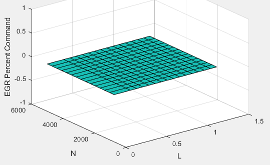Engine speed breakpoints, N, in rpm.

The EGR area percent command, EGRapcmd, lookup table is a function of the normalized mass flow and pressure ratio

`$EGRa{p}_{cmd}={f}_{EGRap,cmd}\left(\frac{{\stackrel{˙}{m}}_{EGRstd,cmd}}{{\stackrel{˙}{m}}_{EGRstd,max}},\frac{{P}_{out,EGR}}{{P}_{in,EGR}}\right)$`

where:

• EGRapcmd is commanded EGR area percent, dimensionless.

• $\frac{{\stackrel{˙}{m}}_{EGRstd,cmd}}{{\stackrel{˙}{m}}_{EGRstd,max}}$ is the normalized mass flow, dimensionless.

• $\frac{{P}_{out,EGR}}{{P}_{in,EGR}}$ is the pressure ratio, dimensionless.Maximum standard EGR valve mass flow breakpoints, ${\stackrel{˙}{m}}_{EGRstd,max}$, in N·m.

Normalized mass flow breakpoints, $\frac{{\stackrel{˙}{m}}_{EGRstd,cmd}}{{\stackrel{˙}{m}}_{EGRstd,max}}$, dimensionless.

Pressure ratio breakpoints, $\frac{{P}_{out,EGR}}{{P}_{in,EGR}}$, dimensionless.

Fuel

Fuel injector slope, ${S}_{inj}$, in mg/ms.

Stoichiometric air-fuel ratio, AFRstoich.

The commanded lambda, ${\lambda }_{cmd}$, lookup table is a function of estimated engine load and measured engine speed

`${\lambda }_{cmd}={f}_{\lambda cmd}\left({L}_{est},N\right)$`

where:

• ${\lambda }_{cmd}$ is commanded relative AFR, dimensionless.

• Lest=L is estimated engine load, dimensionless.

• N is engine speed, in rpm.#### Dependencies

To create this parameter, on the Fuel tab, on the Open-loop fuel pane, clear Input lambda.

#### Dependencies

To create this parameter, on the Fuel tab, on the Open-loop fuel pane, clear Input lambda.

Speed breakpoints, in rpm.

#### Dependencies

To create this parameter, on the Fuel tab, on the Open-loop fuel pane, clear Input lambda.

Engine startup lambda enrichment delta as a function of coolant temperature, dimensionless.

The SI Controller block uses this parameter to account for the extra fuel delivered to the spark-ignition (SI) engine during startup. If the engine speed is greater than the Engine cranking speed parameter, the SI Controller block enriches the optimal relative air-fuel ratio (lambda) with an exponentially decaying delta lambda. To initialize the delta lambda, the block uses the Engine startup lambda enrichment delta vs coolant temperature parameter to create a lambda enrichment table that is a function of the engine coolant temperature. The delta lambda exponentially decays to zero based on a time constant specified with the Engine startup lambda enrichment delta time constant vs coolant temperature parameter.

#### Dependencies

To create this parameter, on the Fuel tab, on the Open-loop fuel pane, clear Input lambda.

Engine startup lambda enrichment delta time constant versus coolant temperature, in s.

The SI Controller block uses this parameter to account for the extra fuel delivered to the spark-ignition (SI) engine during startup. If the engine speed is greater than the Engine cranking speed parameter, the SI Controller block enriches the optimal relative air-fuel ratio (lambda) with an exponentially decaying delta lambda. To initialize the delta lambda, the block uses the Engine startup lambda enrichment delta vs coolant temperature parameter to create a lambda enrichment table that is a function of the engine coolant temperature. The delta lambda exponentially decays to zero based on a time constant specified with the Engine startup lambda enrichment delta time constant vs coolant temperature parameter.

#### Dependencies

To create this parameter, on the Fuel tab, on the Open-loop fuel pane, clear Input lambda.

Engine startup coolant temperature breakpoints, in C.

The SI Controller block uses this parameter to account for the extra fuel delivered to the spark-ignition (SI) engine during startup. If the engine speed is greater than the Engine cranking speed parameter, the SI Controller block enriches the optimal relative air-fuel ratio (lambda) with an exponentially decaying delta lambda. To initialize the delta lambda, the block uses the Engine startup lambda enrichment delta vs coolant temperature parameter to create a lambda enrichment table that is a function of the engine coolant temperature. The delta lambda exponentially decays to zero based on a time constant specified with the Engine startup lambda enrichment delta time constant vs coolant temperature parameter.

#### Dependencies

To create this parameter, on the Fuel tab, on the Open-loop fuel pane, clear Input lambda.

Select option to minimize the commanded air-fuel-ratio (lambda), λcmd error.

#### Dependencies

Selecting this parameter enables these parameters:

• Closed-loop fuel proportional gain, ClsdLpFuelPGain

• Closed-loop fuel integral gain, ClsdLpFuelIGain

• Closed-loop fuel integrator limit, ClsdLpFuelIntgLmt

• Oxygen sensor stoichiometric reset voltage, O2ResetStoichVoltSen

• Oxygen sensor minimum voltage reset, O2ResetMinVoltSen

• Oxygen sensor maximum voltage reset, O2ResetMaxVoltSen

• Oxygen sensor voltage learn update period, O2LearnUpdatePerSen

• Oxygen sensor voltage amplitude minimum, O2AmpMinVoltSen

Configure the block to model dither. For open-loop analysis, select this option to tune for maximum catalytic conversion efficiency.

#### Dependencies

By default, selecting Closed-loop feedback configures the block to model dither.

To enable this parameter for open-loop air-fuel-ratio (lambda) commands, clear Closed-loop feedback.

Selecting this parameter enables these parameters:

Closed-loop fuel proportional gain, dimensionless.

#### Dependencies

To enable this parameter, on the Fuel tab, on the Closed-loop fuel pane, select Closed-loop feedback.

Closed-loop fuel integral gain, dimensionless.

#### Dependencies

To enable this parameter, on the Fuel tab, on the Closed-loop fuel pane, select Closed-loop feedback.

Closed-loop fuel integrator limit, dimensionless.

#### Dependencies

To enable this parameter, on the Fuel tab, on the Closed-loop fuel pane, select Closed-loop feedback.

Lambda dither amplitude, dimensionless.

#### Dependencies

To enable this parameter, on the Fuel tab, on the Closed-loop fuel pane, select either Closed-loop feedback or Dither.

Lambda dither frequency, in Hz.

#### Dependencies

To enable this parameter, on the Fuel tab, on the Closed-loop fuel pane, select either Closed-loop feedback or Dither.

Oxygen sensor stoichiometric reset voltage, O2ResetStoichVoltSen, in mV.

#### Dependencies

To enable this parameter, on the Fuel tab, on the Closed-loop fuel pane, select Closed-loop feedback.

Oxygen sensor minimum voltage reset, O2ResetMinVoltSen, in mV.

#### Dependencies

To enable this parameter, on the Fuel tab, on the Closed-loop fuel pane, select Closed-loop feedback.

Oxygen sensor maximum voltage reset, O2ResetMaxVoltSen, in mV.

#### Dependencies

To enable this parameter, on the Fuel tab, on the Closed-loop fuel pane, select Closed-loop feedback.

Oxygen sensor voltage learn update period, O2LearnUpdatePerSen, in mV.

#### Dependencies

To enable this parameter, on the Fuel tab, on the Closed-loop fuel pane, select Closed-loop feedback.

Oxygen sensor voltage amplitude minimum, O2AmpMinVoltSen, in mV.

#### Dependencies

To enable this parameter, on the Fuel tab, on the Closed-loop fuel pane, select Closed-loop feedback.

#### Dependencies

To enable this parameter, on the Fuel tab, on the Closed-loop fuel pane, select Closed-loop feedback.

#### Dependencies

To enable this parameter, on the Fuel tab, on the Closed-loop fuel pane, select Closed-loop feedback.

Spark

The spark advance lookup table is a function of estimated load and engine speed.

`$SA={f}_{SA}\left({L}_{est},N\right)$`

where:

• Lest=L is estimated engine load, dimensionless.

• N is engine speed, in rpm.Speed breakpoints, in rpm.

Idle Speed

Target idle speed, Nidle, in rpm.

Torque to enable the idle speed controller, Trqidlecmd,enable, in N·m.

Maximum idle controller commanded torque, Trqidlecmd,max, in N·m.

Proportional gain for idle speed control, Kp,idle, in N·m/rpm.

Integral gain for idle speed control, Ki,idle, in N·m/(rpm·s).

Engine speed limit, Nlim, in rpm.

If the engine speed, N, exceeds the engine speed limit, Nlim, the block sets the commanded engine torque to 0.

To smoothly transition the torque command to 0 as the engine speed approaches the speed limit, the block implements a lookup table multiplier. The lookup table multiplies the torque command by a value that ranges from 0 (engine speed exceeds limit) to 1 (engine speed does not exceed the limit).

Engine cranking speed, in rpm.

Stop-Start

Select to enable the engine stop-start logic. Selecting this option will activate additional parameters to modify the behavior of the Engine Stop-Start block.

Select to add a port to the engine controller block which enables or disables the stop-start logic.

#### Dependencies

To enable this parameter, on the Stop-Start tab, select Enable Engine Stop-Start.

Engine stop time for the stop-start logic, in s.

#### Dependencies

To enable this parameter, on the Stop-Start tab, select Enable Engine Stop-Start.

Catalyst light off time for the stop-start logic, in s.

#### Dependencies

To enable this parameter, on the Stop-Start tab, select Enable Engine Stop-Start.

Sample time for the stop-start logic, in s.

#### Dependencies

To enable this parameter, on the Stop-Start tab, select Enable Engine Stop-Start.

### Estimation

Air

Number of engine cylinders, ${N}_{cyl}$.

Crankshaft revolutions per power stroke, $Cps$, in rev/stroke.

Displaced volume, ${V}_{d}$, in m^3.

Ideal gas constant, ${R}_{air}$, in J/(kg·K).

Standard air pressure, ${P}_{std}$, in Pa.

Standard air temperature, ${T}_{std}$, in K.

The engine volumetric efficiency lookup table, ${f}_{{\eta }_{v}}$, is a function of intake manifold absolute pressure and engine speed

`${\eta }_{v}={f}_{{\eta }_{v}}\left(MAP,N\right)$`

where:

• ${\eta }_{v}$ is engine volumetric efficiency, dimensionless.

• MAP is intake manifold absolute pressure, in KPa.

• N is engine speed, in rpm.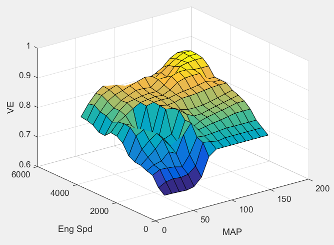#### Dependencies

To enable this parameter, for the Air mass flow estimation model parameter, select `Simple Speed-Density`.

Intake manifold pressure breakpoints for speed-density volumetric efficiency lookup table, in KPa.

#### Dependencies

To enable this parameter, for the Air mass flow estimation model parameter, select `Simple Speed-Density`.

Engine speed breakpoints for speed-density volumetric efficiency lookup table, in rpm.

#### Dependencies

To enable this parameter, for the Air mass flow estimation model parameter, select `Simple Speed-Density`.

The cylinder volume at intake valve close table (IVC), ${f}_{Vivc}$ is a function of the intake cam phaser angle

`${V}_{IVC}={f}_{Vivc}\left({\phi }_{ICP}\right)$`

where:

• ${V}_{IVC}$ is cylinder volume at IVC, in L.

• ${\phi }_{ICP}$ is intake cam phaser angle, in crank advance degrees.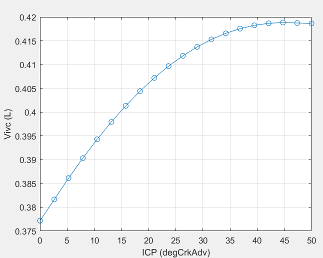#### Dependencies

To enable this parameter, for the Air mass flow estimation model parameter, select ```Dual Variable Cam Phasing```.

Engine speed breakpoints, in rpm.

#### Dependencies

To enable this parameter, for the Air mass flow estimation model parameter, select ```Dual Variable Cam Phasing```.

Cylinder volume at intake valve close table breakpoints.

#### Dependencies

To enable this parameter, for the Air mass flow estimation model parameter, select ```Dual Variable Cam Phasing```.

The trapped mass correction factor table, ${f}_{TMcorr}$, is a function of the normalized density and engine speed

where:

• $T{M}_{corr}$, is trapped mass correction multiplier, dimensionless.

• ${\rho }_{norm}$ is normalized density, dimensionless.

• N is engine speed, in rpm.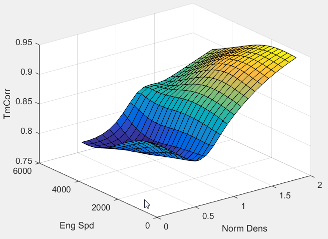#### Dependencies

To enable this parameter, for the Air mass flow estimation model parameter, select ```Dual Variable Cam Phasing```.

Normalized density breakpoints.

#### Dependencies

To enable this parameter, for the Air mass flow estimation model parameter, select ```Dual Variable Cam Phasing```.

The phaser intake mass flow model lookup table is a function of exhaust cam phaser angles and trapped air mass flow

`${\stackrel{˙}{m}}_{intkideal}={f}_{intkideal}\left({\phi }_{ECP},T{M}_{flow}\right)$`

where:

• ${\stackrel{˙}{m}}_{intkideal}$ is engine intake port mass flow at arbitrary cam phaser angles, in g/s.

• ${\phi }_{ECP}$ is exhaust cam phaser angle, in degrees crank retard.

• $T{M}_{flow}$ is flow rate equivalent to corrected trapped mass at the current engine speed, in g/s.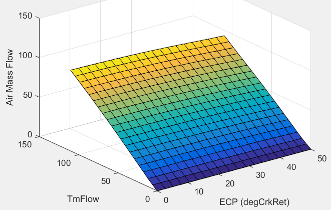#### Dependencies

To enable this parameter, for the Air mass flow estimation model parameter, select ```Dual Variable Cam Phasing```.

Exhaust cam phaser breakpoints for air mass flow lookup table.

#### Dependencies

To enable this parameter, for the Air mass flow estimation model parameter, select ```Dual Variable Cam Phasing```.

Trapped mass flow breakpoints for air mass flow lookup table.

#### Dependencies

To enable this parameter, for the Air mass flow estimation model parameter, select ```Dual Variable Cam Phasing```.

The intake air mass flow correction lookup table, ${f}_{aircorr}$, is a function of ideal load and engine speed

`${\stackrel{˙}{m}}_{air}={\stackrel{˙}{m}}_{intkideal}{f}_{aircorr}\left({L}_{ideal},N\right)$`

where:

• ${L}_{ideal}$ is engine load (normalized cylinder air mass) at arbitrary cam phaser angles, uncorrected for final steady-state cam phaser angles, dimensionless.

• N is engine speed, in rpm.

• ${\stackrel{˙}{m}}_{air}$ is engine intake air mass flow final correction at steady-state cam phaser angles, in g/s.

• ${\stackrel{˙}{m}}_{intkideal}$ is engine intake port mass flow at arbitrary cam phaser angles, in g/s.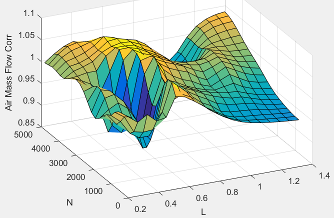#### Dependencies

To enable this parameter, for the Air mass flow estimation model parameter, select ```Dual Variable Cam Phasing```.

Engine load breakpoints for air mass flow final correction.

#### Dependencies

To enable this parameter, for the Air mass flow estimation model parameter, select ```Dual Variable Cam Phasing```.

Engine speed breakpoints for air mass flow final correction.

#### Dependencies

To enable this parameter, for the Air mass flow estimation model parameter, select ```Dual Variable Cam Phasing```.

EGR flow time constant, τEGR, in s.

The pressure ratio is a function of the standard mass flow

`$\frac{{P}_{out,EGR}}{{P}_{amb}}={f}_{intksys,pr}\left({\stackrel{˙}{m}}_{air,std}\right)$`

where:

• ${\stackrel{˙}{m}}_{air,std}$ is standard mass flow, in g/s.

• $\frac{{P}_{out,EGR}}{{P}_{amb}}$ is pressure ratio, dimensionless.Standard mass flow, ${\stackrel{˙}{m}}_{air,std}$, in g/s.

The EGR valve standard mass flow lookup table is a function of EGR valve area percent and the pressure ratio

`${\stackrel{˙}{m}}_{EGR,std}={f}_{EGR,std}\left(EGRap,\frac{{P}_{out,EGR}}{{P}_{in,EGR}}\right)$`

where:

• ${\stackrel{˙}{m}}_{EGR,std}$ is EGR valve standard mass flow, dimensionless.

• EGRap is EGR valve flow area percent, in percent.

• $\frac{{P}_{out,EGR}}{{P}_{in,EGR}}$ is the pressure ratio, dimensionless.EGR valve standard flow pressure ratio,$\frac{{P}_{out,EGR}}{{P}_{in,EGR}}$, dimensionless.

EGR valve flow area percent, EGRap, in percent.

Torque

For the simple torque lookup table model, the SI engine uses a lookup table map that is a function of engine speed and load, ${T}_{brake}={f}_{TnL}\left(L,N\right)$, where:

• ${T}_{brake}$ is engine brake torque after accounting for spark advance, AFR, and friction effects, in N·m.

• L is engine load, as a normalized cylinder air mass, dimensionless.

• N is engine speed, in rpm.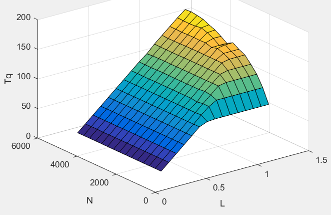The simple torque lookup model assumes that the calibration has negative torque values to indicate the non-firing engine load (L) versus speed (N) condition. The calibrated table (L-by-N) contains the non-firing data in the first table row (1-by-N). When the fuel delivered to the engine is zero, the model uses the data in the first table row (1-by-N) at or above 100 AFR. 100 AFR results from fuel cutoff or very lean operation where combustion cannot occur.

#### Dependencies

To enable this parameter, for the Torque model parameter, select `Simple Torque Lookup`.

#### Dependencies

To enable this parameter, for the Torque model parameter, select `Simple Torque Lookup`.

Engine speed breakpoints, N, in rpm.

#### Dependencies

To enable this parameter, for the Torque model parameter, select `Simple Torque Lookup`.

If you select Crank angle pressure and torque on the block Torque tab, you can:

• Simulate advanced closed-loop engine controls in desktop simulations and on HIL bench, based on cylinder pressure recorded from a model or laboratory test as a function of crank angle.

• Simulate driveline vibrations downstream of the engine due to high-frequency crankshaft torsionals.

• Simulate engine misfires due to lean operation or spark plug fouling by using the injector pulse width input.

• Simulate cylinder deactivation effect (closed intake and exhaust valves, no injected fuel) on individual cylinder pressures, mean-value airflow, mean-value torque, and crank-angle-based torque.

• Simulate the fuel-cut effect on individual cylinder pressure, mean-value torque, and crank-angle-based torque.

#### Dependencies

To enable this parameter, set Torque model to `Torque Structure`.

Cylinder pressure table Prs, as a function of speed N, load L, and crank angle M, in Pa.

#### Dependencies

To enable this parameter, for the Torque model parameter, select `Torque Structure`. Select Crank angle pressure and torque.

Brake torque table Tbrake, as a function of speed N, load L, and crank angle M, in N·m.

#### Dependencies

To enable this parameter, for the Torque model parameter, select `Torque Structure`. Select Crank angle pressure and torque.

Speed breakpoints, N, in rpm.

#### Dependencies

To enable this parameter, for the Torque model parameter, select `Torque Structure`. Select Crank angle pressure and torque.

#### Dependencies

To enable this parameter, for the Torque model parameter, select `Torque Structure`. Select Crank angle pressure and torque.

Crank angle breakpoints, M, in deg.

#### Dependencies

To enable this parameter, for the Torque model parameter, select `Torque Structure`. Select Crank angle pressure and torque.

Top dead center (TDC) compression angles by cylinder, in deg.

#### Dependencies

To enable this parameter, for the Torque model parameter, select `Torque Structure`. Select Crank angle pressure and torque.

The inner torque lookup table, ${f}_{Tqinr}$, is a function of engine speed and engine load, $T{q}_{inr}={f}_{Tqinr}\left(L,N\right)$, where:

• $T{q}_{inr}$ is inner torque based on gross indicated mean effective pressure, in N·m.

• L is engine load at arbitrary cam phaser angles, corrected for final steady-state cam phaser angles, dimensionless.

• N is engine speed, in rpm.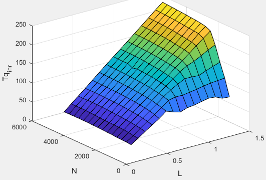#### Dependencies

To enable this parameter, for the Torque model parameter, select `Torque Structure`.

The friction torque lookup table, ${f}_{Tfric}$, is a function of engine speed and engine load, ${T}_{fric}={f}_{Tfric}\left(L,N\right)$, where:

• ${T}_{fric}$ is friction torque offset to inner torque, in N·m.

• L is engine load at arbitrary cam phaser angles, corrected for final steady-state cam phaser angles, dimensionless.

• N is engine speed, in rpm.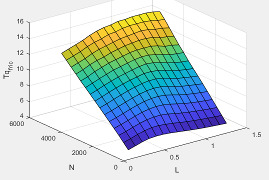#### Dependencies

To enable this parameter, for the Torque model parameter, select `Torque Structure`.

Engine temperature modifier on friction torque, ƒfric,temp, dimensionless.

#### Dependencies

To enable this parameter, for the Torque model parameter, select `Torque Structure`.

Engine temperature modifier breakpoints, in K.

#### Dependencies

To enable this parameter, for the Torque model parameter, select `Torque Structure`.

The pumping work lookup table, ƒTpump, is a function of engine load and engine speed, `Tpump=ƒTpump(L,N)`, where:

• Tpump is pumping work, in N·m.

• L is engine load, as a normalized cylinder air mass, dimensionless.

• N is engine speed, in rpm.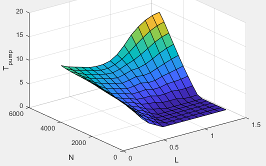#### Dependencies

To enable this parameter, for the Torque model parameter, select `Torque Structure`.

The optimal spark lookup table, ${f}_{SAopt}$, is a function of engine speed and engine load, $S{A}_{opt}={f}_{SAopt}\left(L,N\right)$, where:

• SAopt is optimal spark advance timing for maximum inner torque at stoichiometric air-fuel ratio (AFR), in deg.

• L is engine load at arbitrary cam phaser angles, corrected for final steady-state cam phaser angles, dimensionless.

• N is engine speed, in rpm.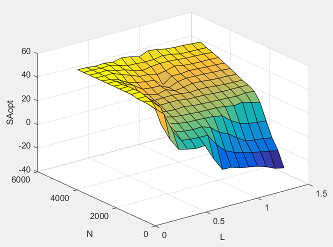#### Dependencies

To enable this parameter, for the Torque model parameter, select `Torque Structure`.

#### Dependencies

To enable this parameter, for the Torque model parameter, select `Torque Structure`.

Inner torque speed breakpoints, in rpm.

#### Dependencies

To enable this parameter, for the Torque model parameter, select `Torque Structure`.

The spark efficiency lookup table, ${f}_{Msa}$, is a function of the spark retard from optimal

`$\begin{array}{l}{M}_{sa}={f}_{Msa}\left(\Delta SA\right)\\ \Delta SA=S{A}_{opt}-SA\end{array}$`

where:

• ${M}_{sa}$ is the spark retard efficiency multiplier, dimensionless.

• $\Delta SA$is the spark retard timing distance from optimal spark advance, in deg.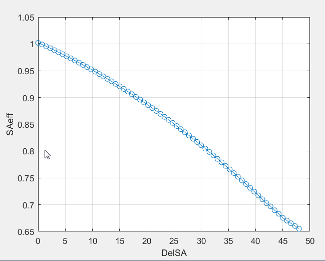#### Dependencies

To enable this parameter, for the Torque model parameter, select `Torque Structure`.

Spark retard from optimal inner torque timing breakpoints, in deg.

#### Dependencies

To enable this parameter, for the Torque model parameter, select `Torque Structure`.

The lambda efficiency lookup table, ${f}_{M\lambda }$, is a function of lambda, ${M}_{\lambda }={f}_{M\lambda }\left(\lambda \right)$, where:

• ${M}_{\lambda }$ is the lambda multiplier on inner torque to account for the air-fuel ratio (AFR) effect, dimensionless.

• $\lambda$ is lambda, AFR normalized to stoichiometric fuel AFR, dimensionless.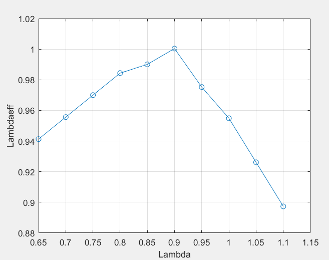#### Dependencies

To enable this parameter, for the Torque model parameter, select `Torque Structure`.

Lambda effect on inner torque lambda breakpoints, dimensionless.

#### Dependencies

To enable this parameter, for the Torque model parameter, select `Torque Structure`.

Exhaust

The exhaust temperature lookup table, ${f}_{Texh}$, is a function of engine load and engine speed

`${T}_{exh}={f}_{Texh}\left(L,N\right)$`

where:

• Texh is engine exhaust temperature, in K.

• L is normalized cylinder air mass or engine load, dimensionless.

• N is engine speed, in rpm.Engine load breakpoints used for exhaust temperature lookup table.

Engine speed breakpoints used for exhaust temperature lookup table, in rpm.

 Gerhardt, J., Hönninger, H., and Bischof, H., A New Approach to Functional and Software Structure for Engine Management Systems — BOSCH ME7. SAE Technical Paper 980801, 1998.

 Heywood, John B. Internal Combustion Engine Fundamentals. New York: McGraw-Hill, 1988.

 Leone, T. Christenson, E., Stein, R., Comparison of Variable Camshaft Timing Strategies at Part Load. SAE Technical Paper 960584, 1996, doi:10.4271/960584.

 Liu, F. and Pfeiffer, J., Estimation Algorithms for Low Pressure Cooled EGR in Spark-Ignition Engines. SAE Int. J. Engines 8(4):2015, doi:10.4271/2015-01-1620.